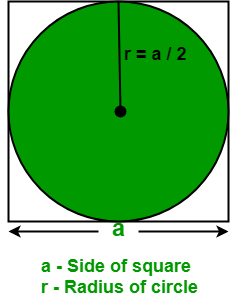# Program to calculate area of an Circle inscribed in a Square

Given the side of a square. The task is to find the area of an inscribed circle in a square.

Examples:

Input : a = 8
Output : Area of an inscribed circle: 50.24

Input : a = 12.04
Output : Area of an inscribed circle: 113.795


## Recommended: Please try your approach on {IDE} first, before moving on to the solution.

Given a square i.e. all sides of a square are of equal length and all four angles are 90 degrees. Below diagram depicts an inscribed circle in a square.Properties of an inscribed circle in a square:

• The diameter of an inscribed circle in a square is equal to the length of the side of a square.
• With at least one measure of the circle or the square, the area and the perimeter of the square can be calculated in which the circle is inscribed.
• The center of the square and the center of the circle lie at a same point.
• when at least one measure of the circle or the square is given, the circumference and area of the circle can be calculated.
• Formula to find the area of an inscribed circle:where a is the side of a square in which a circle is inscribed.

How does the formula works?
Assume a is the side of a square and we know that a square has 4 sides.

Area of a circle =where r is the radius of a circle and area of a square = a2

Therefore, the area of an inscribed circle in a square =Now, put r = a / 2
So, the area of an inscribed circle in a square =## C++

 // C++ Program to find the area of   // an inscribed circle in a square.  #include  #define PI 3.14  using namespace std;     // Function to find area of an   // inscribed circle in a square.  float areaOfInscribedCircle(float a)  {      return ( PI / 4 ) * a * a;  }     // Driver's code  int main()  {      float a = 8;             cout << "Area of an inscribed circle: "          << areaOfInscribedCircle(a);                  return 0;  }

## Java

 // Java Program to find the area of   // an inscribed circle in a square.  import java.io.*;     class GFG {             static double PI = 3.14;             // Function to find area of an       // inscribed circle in a square.      static double areaOfInscribedCircle(float a)      {          return ( PI / 4 ) * a * a;      }         // Driver code      public static void main (String[] args)      {          float a = 8;                 System.out.println("Area of an inscribed"         + " circle: " + areaOfInscribedCircle(a));      }  }

## Python 3

 # Python Program to find the area of   # an inscribed circle in a square.         PI = 3.14        # Function to find area of an   # inscribed circle in a square.  def areaOfInscribedCircle(a):      return ( PI / 4 ) * a * a     # Driver code  a = 8 print("Area of an inscribed circle:",    round(areaOfInscribedCircle(a), 2))

## C#

 // C# Program to find the   // area of an inscribed   // circle in a square.  using System;     class GFG   {      static double PI = 3.14;             // Function to find area        // of an inscribed circle      // in a square.      static double areaOfInscribedCircle(float a)      {          return (PI / 4 ) * a * a;      }         // Driver code      public static void Main ()      {          float a = 8;                 Console.WriteLine("Area of an inscribed" +                                        " circle: " +                          areaOfInscribedCircle(a));      }  }     // This code is contributed  // by anuj_6

## PHP

 

Output:

Area of an inscribed circle:50.24


Attention reader! Don’t stop learning now. Get hold of all the important DSA concepts with the DSA Self Paced Course at a student-friendly price and become industry ready.

My Personal Notes arrow_drop_upCheck out this Author's contributed articles.

If you like GeeksforGeeks and would like to contribute, you can also write an article using contribute.geeksforgeeks.org or mail your article to contribute@geeksforgeeks.org. See your article appearing on the GeeksforGeeks main page and help other Geeks.

Please Improve this article if you find anything incorrect by clicking on the "Improve Article" button below.

Improved By : vt_m

Article Tags :
Practice Tags :

Be the First to upvote.

Please write to us at contribute@geeksforgeeks.org to report any issue with the above content.